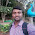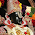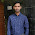## Friday, September 25, 2015

### DC Machine Multiple Choice Questions with Solutions: Part-12

DC Motor DC Generator Multiple Choice Questions with Solutions:

 Which of the following statements is false?
(a) A DC motor converts electrical energy to mechanical energy
(b) The efficiency of a DC motor is the ratio input power to output power
(c) A DC generator converts mechanical power to electrical power
(d) The efficiency of a DC generator is the ratio output power to input power
Options:
1. C only
2. C and D only
3. B only
4. None of the above

 If the speed of a DC machine is doubled and the flux remains constant, the generated e.m.f.
(a) remains the same
(b) is doubled
(c) is halved
(d) None of the above

 If the flux per pole of a shunt-wound DC generator is increased, and all other variables are kept the same, the speed
(a) decreases
(b) stays the same
(c) increases
(d) None of the above

 Which of the following statements is false?
(a) A commutator is necessary as part of a DC motor to keep the armature rotating in the same direction
(b) A commutator is necessary as part of a DC generator to produce unidirectional voltage at the terminals of the generator
(c) The field winding of a DC machine is housed in slots on the armature
(d) The brushes of a DC machine are usually made of carbon and do not rotate with the armature
Options:
1. D only
2. C and D only
3. None of the above
4. C only

 If the flux per pole of a shunt-wound DC generator is halved, the generated e.m.f. at constant speed
(a) is doubled
(b) is halved
(c) remains the same
(d) None of the above

 In a series-wound generator running at constant speed, as the load current increases, the terminal voltage
(a) increases
(b) decreases
(c) stays the same
(d) None of the above

 Which of the following statements is false for a series-wound DC motor?
(a) The speed decreases with increase of resistance in the armature circuit
(b) The speed increases as the flux decreases
(c) The speed can be controlled by a diverter
(d) The speed can be controlled by a shunt field regulator

 The armature resistance of a DC motor is 0.5 , the supply voltage is 200V and the back e.m.f. is 196V at full speed. The armature current is:
(a) 4A
(b) 8A
(c) 400A
(d) 392A

 In DC generators iron losses are made up of:
(a) hysteresis and friction losses
(b) hysteresis, eddy current and brush contact losses
(c) hysteresis and eddy current losses
(d) hysteresis, eddy current and copper losses

 The effect of inserting a resistance in series with the field winding of a shunt motor is to:
(a) increase the magnetic field
(b) increase the speed of the motor
(c) decrease the armature current
(d) reduce the speed of the motor

Thanks for reading... Please like us in the facebook and subscribe to get new posts to your mail id...
1:07 AM

1.Nice. Very useful. Thanks

2.3.Please upload more questions on d.c. machine chapter.

4.Add some more questions....

5.Nice keep doing
Improve trust

6.For mathematical questions plz give expations

7.Need more question.

8.Nice. I got 9

9.Very useful for us

10.Very useful Thanks

11.12.13.14.Pls add explanation to theory qns

15.16.VERY EASY TO UNDERSTAND.

17.VERY EASY TO UNDERSTAND.

18.ANKITA NOVEMBER,27 AT EVENINGNovember 27, 2016 at 9:10 AM

19.20.its really useful for interviews

21.22.add formulas And expalaination

23.send me some useful formulas in electrical engg, and some sample question papers of KPTCL

24.Anonymous... the answer for Question No.6 is A only ---> series machine the flux is directly proportional to armature current as current increases (i.e;flux increases) which in turn to maintain speed as constant to increase the voltage

25.Anonymous... the answer for Question No.6 is A only ---> series machine the flux is directly proportional to armature current as current increases (i.e;flux increases) which in turn to maintain speed as constant to increase the voltage

26.The ri8 answer for 6th is b